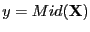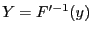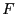Next: Kantorovitch theorem Up: Moore theorem Previous: Mathematical background   Contents

Implementation

The previous test is implemented forand. The procedure is implemented as:

int Krawczyk_Analyzer(int m,int n,
INTERVAL_VECTOR (* IntervalFunction)(int,int,INTERVAL_VECTOR &),
INTERVAL_MATRIX (* J)(int, int, INTERVAL_VECTOR &),INTERVAL_VECTOR &Input)
with
• m: the number of unknown
• n: the number of equations
• IntervalFunction: a function which return the interval vector evaluation of the equations, see note 2.3.4.3
• J: a function which calculate an interval evaluation of the elements of the jacobian of the equations, see note 2.4.2.2
• Input: the ranges for the variables
This procedure returns 1 if there is a unique solution ofin Input.

Jean-Pierre Merlet 2012-12-20